Next: Meridian Transits Up: Spherical Astronomy Previous: Local Horizon and Meridian

# Horizontal Coordinates

It is convenient to specify the positions of celestial objects in the sky, when viewed from a particular observation site,, on the earth's surface, using a pair of angular coordinates,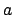and, which are measured with respect to the local horizon. Let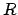denote a celestial object, and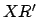the projection of the line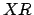onto the horizontal plane,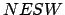--see Fig. 11. The coordinate, which is known as altitude, is the angle subtended betweenand. Objects above the horizon have positive altitudes, whereas objects below the horizon have negative altitudes. The zenith has altitude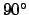, and the horizon altitude. The coordinate, which is known as azimuth, is the angle subtended between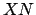and. Azimuth increases from the north towards the east. Thus, the north, east, south, and west compass points have azimuths of,,, and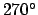, respectively. Note that the basis vectors in the horizontal coordinate system are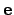,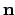, and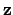, whereas the corresponding basis vectors in the celestial coordinate system are,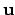, and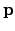--see Figs. 3 and 8. By analogy with Eqs. (1)--(3), we can write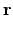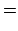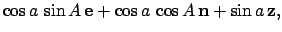(27)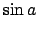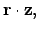(28)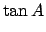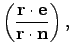(29)

where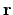is a unit vector directed fromto. Hence, it follows from Eqs. (1), and (22)-(23), that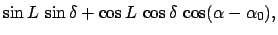(30)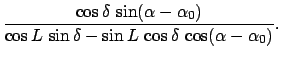(31)

These expressions allow us to calculate the altitude and azimuth of a celestial object of declination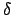and right ascension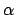which is viewed from an observation site on the earth's surface of terrestrial latitude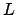at an instant in time when celestial objects of right ascension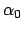are culminating at the meridian. According to Eqs. (8), and (25)-(26), the altitude and azimuth of a similarly viewed point on the ecliptic (i.e.,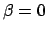) of ecliptic longitude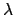are given by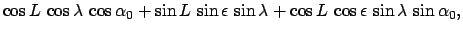(32)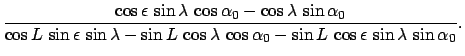(33)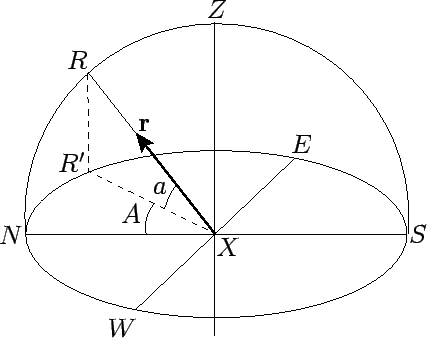Next: Meridian Transits Up: Spherical Astronomy Previous: Local Horizon and Meridian
Richard Fitzpatrick 2010-07-21